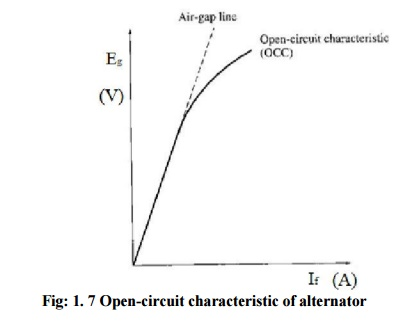Home | | Electrical Machines II | Open circuit characteristic (OCC) and Short Circuit Characteristics (SCC) of a Synchronous generator

# Open circuit characteristic (OCC) and Short Circuit Characteristics (SCC) of a Synchronous generator

With the armature terminals open, Ia=0, so Eg = Vt It is thus possible to construct a plot of Eg or Vt vs If graph.

## Open-circuit characteristic (OCC) of a generator

With the armature terminals open, Ia=0, so Eg = Vt It is thus possible to construct a plot of Eg or Vt vs If graph. This plot is called open-circuit characteristic (OCC) of a generator. With this characteristic, it is possible to find the internal generated voltage of the generator for any given field current.Initially OCC follows a straight-line relation with the field current as long as the magnetic circuit of the synchronous generator does not saturate. This straight line is appropriately called the air-gap line. Practically due to saturation induced emf bend from the straight line.

## Short Circuit Characteristics (SCC)For getting SCC generator is rotated at rated speed with armature terminals short circuited. The field current is adjusted to 0. The armature current is measured as the field current is increased.

Study Material, Lecturing Notes, Assignment, Reference, Wiki description explanation, brief detail
Electrical machines : Synchronous Generator : Open circuit characteristic (OCC) and Short Circuit Characteristics (SCC) of a Synchronous generator |